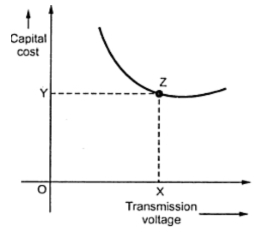Economic Choice of Transmission Voltage

The cost of conductor material required can be reduced with reduction in volume of conduction           material which is possible with increase in transmission voltage. The volume of conductor material is inversely proportional to transmission voltage as seen in previous posts. So it may be economical from point of view of cost of conductor material to go for maximum possible transmission voltage.
But with increase in transmission voltage, there will be corresponding increase in cost of insulators, transformers, switchgear and other equipments. Thus for overall economy, there is optimum transmission voltage. The economical transmission voltage is one for which cost of conductor, insulators, transformers, switchgear and other equipments is minimum.
If the power to be transmitted, generation voltage and length of transmission are known quantities, then economical transmission voltage can be computed. Initially some standard transmission voltage is selected and costs of transformers, switchgear, conductors and other equipments are determined.
The transformers are present at generating and receiving ends of transmission line. With increase in voltage for a given power, the cost of transformer rises. Also the cost of switchgear, lightning arrester, insulation and supports increases while the cost of conductors decreases with increase in voltage. The total cost of transmission line for a given voltage is sum of costs of transformers, switchgear, lightning  arrester, insulators, support and conductors. These costs and total cost is also computed for different transmission voltages. From this data, a graph is drawn between total cost of transmission line versus transmission voltage which is shown in Fig. 1.Fig. 1

The economical voltage is one for which capital cost associated with line is minimum which is point Z from the Fig. 1. Thus optimum transmission voltage is represented by OX.
The application of this method is practice is rare as various costs associated in it can not be determined with a good degree of accuracy. Instead, an empirical formula is used for finding economical voltage. Thus the economic voltage between lines in 3 phase system is given by,

Here V = Line voltage in KV, L = Distance of transmission line in Km, P = Maximum power per phase to be delivered to single circuit.
The economical transmission voltage depends on length (or distance) of transmission line and power to be transmitted. With increase in distance of transmission line, the cost of equipments and apparatus increases which results in higher transmission voltage. If the power to be transmitted is large then large units of generating and transforming are required which reduces the cost per Kw of terminal equipments.

1 comment:

1.Large transmission line material configurations with high voltage and current levels generate large values of electric and magnetic fields stresses which affect the human being and the nearby objects located at ground surfaces.